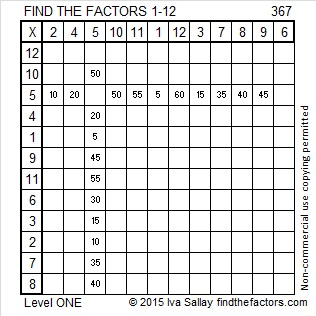# 367 and Level 1

2^367 (mod 367) = 2, so 367 is VERY LIKELY a prime number. Scroll down below the puzzle to know for sure.Print the puzzles or type the factors on this excel file:12 Factors 2015-01-26

• 367 is a prime number.
• Prime factorization: 367 is prime.
• The exponent of prime number 367 is 1. Adding 1 to that exponent we get (1 + 1) = 2. Therefore 367 has exactly 2 factors.
• Factors of 367: 1, 367
• Factor pairs: 367 = 1 x 367
• 367 has no square factors that allow its square root to be simplified. √367 ≈ 19.157

How do we know that 367 is a prime number? If 367 were not a prime number, then it would be divisible by at least one prime number less than or equal to √367 ≈ 19.157. Since 367 cannot be divided evenly by 2, 3, 5, 7, 11, 13, 17, or 19, we know that 367 is a prime number.